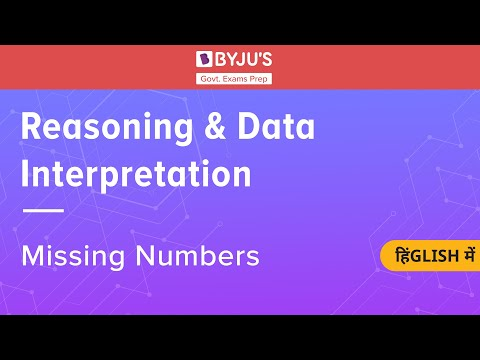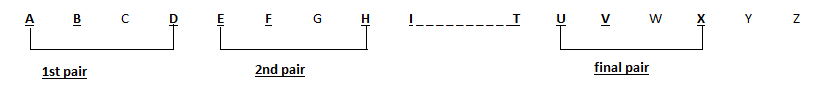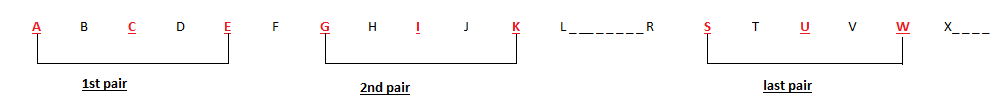# Alphanumeric Series in Reasoning for Government Exams

The reasoning section is one common section which is a part of all major competitive exams conducted in the country. The Alphanumeric series is an important topic in this section.

In this article, we bring you some simple tricks and tips to solve the alphanumeric questions quicker and more efficiently, along with some sample questions related to this topic.

Candidates can prepare for the logical reasoning section by solving more and more questions based on various topics. To get logical reasoning questions, visit the linked article and ace the reasoning ability section.

 Ace the Reasoning Ability questions in the upcoming Government exams by practising more! Refer to the links given below for assistance:

### What is an Alphanumeric Series?

As the name suggests, Alpha-numeric, a series which is formed by combining both alphabets and numbers is called an alphanumeric series. Questions from this topic are mostly asked in the preliminary phase of all Government exams.

The questions from this topic are easy to solve, and candidates can expect at least 1-3 questions from this topic to be asked in the exam.

Visit the alphanumeric series questions page and solve the sample questions based on the topic. Also, this will help strengthen your command over the alphanumeric series concept.## Tips & Tricks To Solve Alphanumeric Questions

There are a variety of types in which questions may be asked for the alphanumerical series. These include:

• Missing Series: Where a series of alphabets and numbers may be given a particular pattern and candidates may be asked to fill in the blank space with the required element
• Alphabet-Number-Symbol: These questions may also be asked in a format where a series with alphabets, number and different types of symbols (like &, *, \$, etc.) may be given, and questions may be asked based on them
• Only Alphabetic Series: Series with either missing element or questions with sub-parts may be asked which comprise only English Alphabets and no numbers, set in a specific pattern
• Only Numerical Series: Series comprising only numbers, set in a specific pattern may be asked the exam. Questions based on this type may be asked in the form of rearrangement of numbers or digits with the number in ascending, descending or any other order.

Candidates can check the detailed logical reasoning syllabus along with some sample questions related to the same at the linked article.

Given below are a few tips and tricks that may help you solve the questions based on the alphanumeric series:

• In pattern-based series, first, analyse alphanumeric series given to you and try to crack the pattern that is being followed. Mostly the pattern may involve change is succeeding or preceding terms of either the number or the alphabet
• In abrupt series, which include number-symbol-alphabet, mostly no pattern is followed. Candidates need to move to the sub-part of the questions and straightaway answer them
• Ensure that for missing number series, you recheck the entire series after finding an answer, to ensure that no error has been made

Aspirants who are preparing for the upcoming Government exams can also refer to the links given below and ace the reasoning ability section:

Another type of questions which may be asked in the form of a number series may comprise numbers arranged both vertically and horizontally and one of element would be missing. Candidates can learn more about these questions and tips to solve them in the video given below:### Solved Examples – Alphanumeric Series

The more an aspirant practise, his/her command over the topic will get stronger and also the understanding of the topic will get better.

So, to make the candidates understand the concept of alphanumeric series and to provide the candidates with few examples to prepare for the exam, given below are a few solved examples.

Directions (Q1-Q2): Refer to the alphanumeric series given below and answer the following questions:

A * 5 9 I N & E @ # U 1 &

Q 1. How many vowels in the above arrangement are preceded by a symbol?

1. One
2. Two
3. Three
4. None
5. Four

Answer: (2) Two; “E” is preceded by “&” and “U” is preceded by “#”

Q 2. Which is the second element to the left of the sixth element from the right?

1. &
2. @
3. N
4. E
5. 9

Answer: (3) N; Sixth element from the right is E and second to its left is N

Directions(Q3-Q4): Find the missing element in the series given below:

Q 3.

ABD EFH IJL MNP QRT ?

1. XYZ
2. VWY
3. WXZ
4. UVX
5. XZA

Solution:Q 4.

ACE ? MOQ     SUW

1. GIK
2. FHJ
3. GHJ
4. GIL
5. EFH

Solution:Directions (Q5-Q6): Refer to the numerical series given below and answer the following questions:

435 224 786 823 902

Q 5. When all the digits in each of the given numbers are arranged in ascending order, which number becomes the highest?

1. 435
2. 224
3. 786
4. 823
5. 902

Solution: When the digits are arranged in ascending order within the number, the new numbers formed are:

435 – 345

224 – 224

786 – 678

823 – 238

902 – 092

Q 6. When the digits within the number are multiplied with each other, the product of which number is the lowest?

1. 435
2. 224
3. 786
4. 823
5. 902

Answer: (5)902; any number multiplied with 0 will give the answer 0, which is the least.

Directions (Q7-Q8): Based on the digit-alphabets-symbols series given below, answer the following questions:

@ 1 8 H L I 6 K * & ? U E

Q 7. How many such consonants are there in the series which are immediately followed by a symbol and preceded by a number?

1. One
2. Two
3. Three
4. None
5. Four

Answer: (1) One; K is followed by “*” and preceded by “6”

Q 8. How many numbers are there in the series which are preceded by a vowel and succeeded by a consonant?

1. Two
2. Four
3. Three
4. None
5. One

Answer: (5) One; 6 is preceded by “I” and succeeded by “K”

The questions given above shall help you understand the concept better and also to understand the type of questions that may be asked in the upcoming competitive exams with respect to this topic.

Also, for candidates who are looking for study for preparation tips regarding other subjects which are part of the Government exams, can refer to the links given below:

It is important that a candidate dedicates equal time in preparation for each subject as for most exams, there are sections cut off along with overall cut off.

Aspirants wishing to get any further assistance regarding the syllabus, exam information, study material or preparation tips can turn to BYJU’S for help.